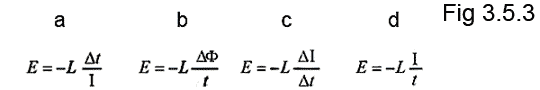# Inductors Quiz

This is a quiz based on the information you can find in Module 3. Hopefully it will be simple. Submit your answers but don't be disappointed if you get answers wrong. Just follow the hints to find the right answer and learn more about inductors.

### 1.What type of is inductor illustrated in Fig 3.5.1 ?

### 2.

The inductance of an inductor will be affected by which property or properties of the inductor's core?

### 3.

Which of the following ranges of inductance values would be most commonly encountered in electronic circuits?

### 4.

Which of the following properties of an inductor change with the applied frequency:

### 5.

What is the value of the inductor shown in Fig 3.5.2?### 6.

Which of the following describes what happens to a non-moving conductor within a changing magnetic field?

### 7.

Which of the formulae shown in Fig 3.5.3 would be used for calculating back e.m.f. ?### 8.

Complete the following statement:
The magnetic field produced by a solenoid shaped inductor is:

### 9.

When using the right hand curl rule for a solenoid, what do the fingers of the right hand indicate?

### 10.

What would be the value of back e.m.f induced at switch off, in a 3.3mH inductor passing a current of 250mA, assuming the time for the current to decay to zero was 50µs?

Top of Page.>